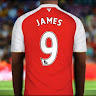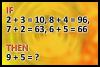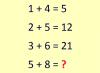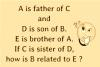BRAIN TEASERSBrain Teasers User Profile

# SA James

rank
187
points
30
See full ranking list
short ranking list
 185 jim white 31 186 Ali Monita Coicá 31 187 SA James 30 188 Shatheesh Chandramohan 29 189 Natasa Ilkic 29
 What is 9 + 5? If 2+3=10, 8+4=96, 7+2=63, 6+5=66 then 9+5=?Calculate 5+8 If 1+4=5, 2+5=12 and 3+6=21 then 5+8=?A is father of C and D is so... A is father of C and D is son of B. E is brother of A. If C is sister of D, how is B related to E?